SPSS TUTORIALS BASICS DATA ANALYSIS T-TEST ANOVA CHI-SQUARE TEST

# Which Statistical Test Should I Use?

## Summary

Finding the appropriate statistical test is easy if you're aware of

1. the basic type of test you're looking for and
2. the measurement levels of the variables involved.

This tutorial briefly defines the 6 basic types of tests and illustrates them with simple examples. We'll then present full overviews of all tests belonging to each type.

## 1. Univariate Tests

Univariate tests are tests that involve only 1 variable. Univariate tests either test if

1. some population parameter -usually a mean or median- is equal to some hypothesized value or
2. some population distribution is equal to some function, often the normal distribution.

A textbook example is a one sample t-test: it tests if a population mean -a parameter- is equal to some value x. This test involves only 1 variable (even if there's many more in your data file).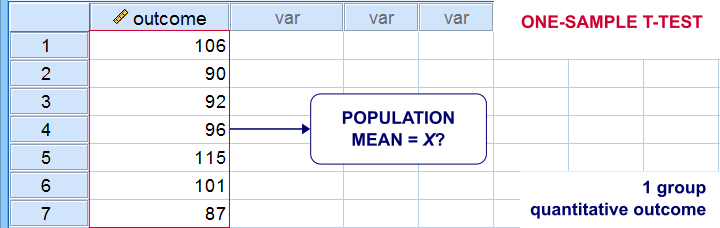## Overview Univariate Tests

MEASUREMENT LEVELNULL HYPOTHESISTEST
DichotomousPopulation proportion = x?Binomial test
Z-test for 1 proportion
CategoricalPopulation distribution = f(x)?Chi-square goodness-of-fit test
QuantitativePopulation mean = x?One-sample t-test
Population median = x?Sign test for 1 median
Population distribution = f(x)?Kolmogorov-Smirnov test
Shapiro-Wilk test

## 2. Within-Subjects Tests

Within-subjects tests compare 2+ variables
measured on the same subjects (often people).
An example is repeated measures ANOVA: it tests if 3+ variables measured on the same subjects have equal population means.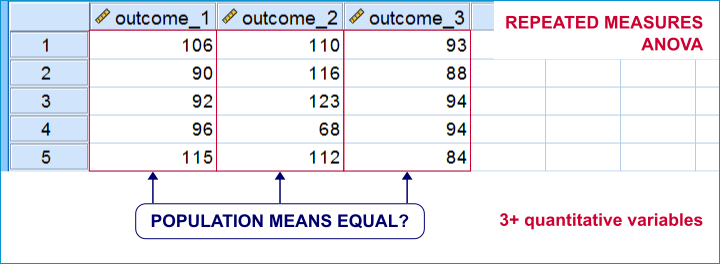Within-subjects tests are also known as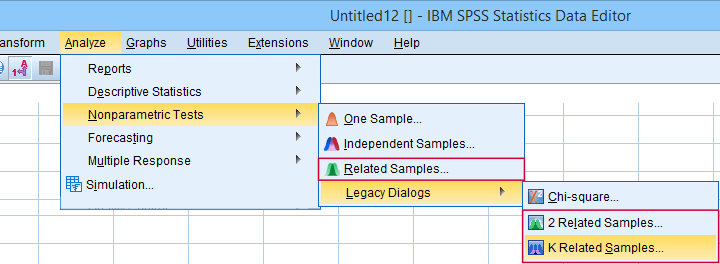“Related samples” refers to within-subjects and “K” means 3+.

## Overview Within-Subjects Tests

MEASUREMENT LEVEL2 VARIABLES3+ VARIABLES
DICHOTOMOUSMcNemar testCochran Q test
ORDINALWilcoxon signed-ranks test
Sign test for 2 related medians
Friedman test
QUANTITATIVEPaired samples t-testRepeated measures ANOVA

## 3. Between-Subjects Tests

Between-subjects tests examines if 2+ subpopulations
are identical with regard to

The best known example is a one-way ANOVA as illustrated below. Note that the subpopulations are represented by subsamples -groups of observations indicated by some categorical variable.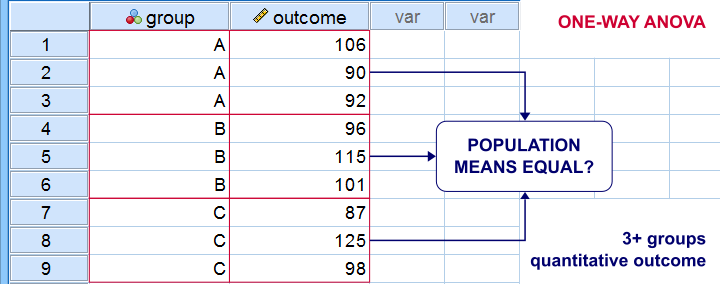“Between-subjects” tests are also known as “independent samples” tests, such as the independent samples t-test. “Independent samples” means that subsamples don't overlap: each observation belongs to only 1 subsample.

## Overview Between-Subjects Tests

OUTCOME VARIABLE2 SUBPOPULATIONS3+ SUBPOPULATIONS
DichotomousZ-test for 2 independent proportionsChi-square independence test
NominalChi-square independence testChi-square independence test
OrdinalMann-Whitney test (mean ranks)
Median test for 2+ independent medians
Kruskal-Wallis test (mean ranks)
Median test for 2+ independent medians
QuantitativeIndependent samples t-test (means)
Levene's test (variances)
One-way ANOVA (means)
Levene's test (variances)

## 4. Association Measures

Association measures are numbers that indicate
to what extent 2 variables are associated.
The best known association measure is the Pearson correlation: a number that tells us to what extent 2 quantitative variables are linearly related. The illustration below visualizes correlations as scatterplots.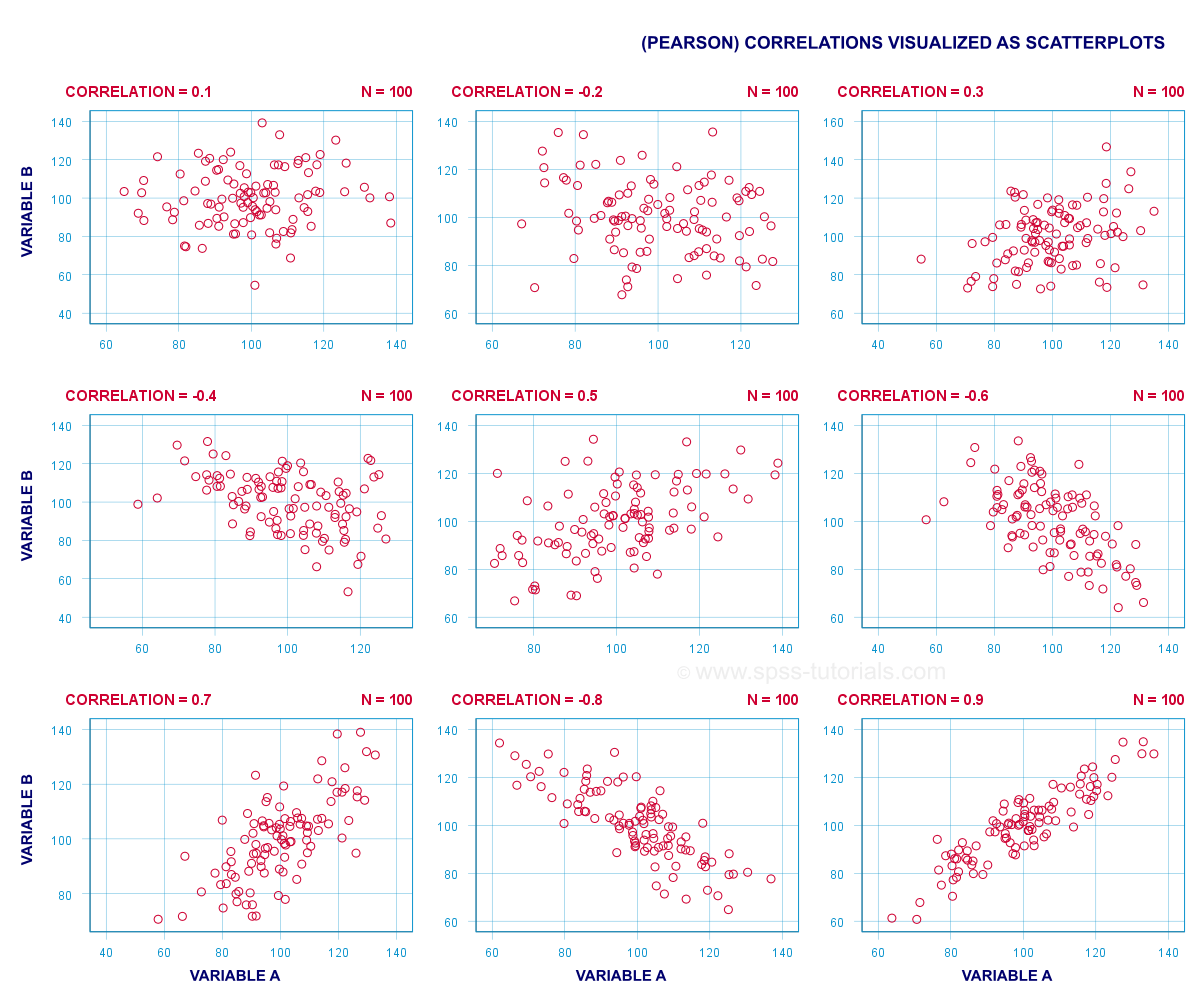## Overview Association Measures

(VARIABLES ARE) QUANTITATIVEORDINALNOMINALDICHOTOMOUS
QUANTITATIVEPearson correlation
ORDINALSpearman correlation
Kendall’s tau
Polychoric correlation
Spearman correlation
Kendall’s tau
Polychoric correlation
NOMINALEta squaredCramér’s V Cramér’s V
DICHOTOMOUSPoint-biserial correlation
Biserial correlation
Spearman correlation
Kendall’s tau
Polychoric correlation
Cramér’s V Phi-coefficient
Tetrachoric correlation

## 5. Prediction Analyses

Prediction tests examine how and to what extent
a variable can be predicted from 1+ other variables.
The simplest example is simple linear regression as illustrated below.Prediction analyses sometimes quietly assume causality: whatever predicts some variable is often thought to affect this variable. Depending on the contents of an analysis, causality may or may not be plausible. Keep in mind, however, that the analyses listed below don't prove causality.

## Overview Prediction Analyses

OUTCOME VARIABLEANALYSIS
Quantitative(Multiple) linear regression analysis
OrdinalDiscriminant analysis or ordinal regression analysis
NominalDiscriminant analysis or nominal regression analysis
DichotomousLogistic regression

## 6. Classification Analyses

Classification analyses attempt to identify and
describe groups of observations or variables.
The 2 main types of classification analysis are

• factor analysis for finding groups of variables (“factors”) and
• cluster analysis for finding groups of observations (“clusters”).

Factor analysis is based on correlations or covariances. Groups of variables that correlate strongly are assumed to measure similar underlying factors -sometimes called “constructs”. The basic idea is illustrated below.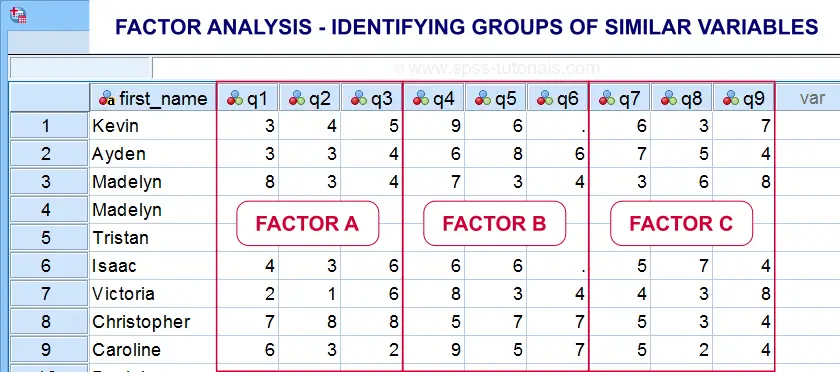Cluster analysis is based on distances among observations -often people. Groups of observations with small distances among them are assumed to represent clusters such as market segments.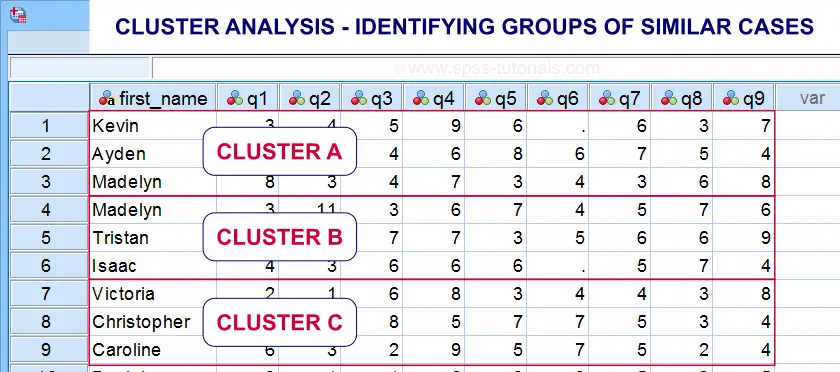Right. So that'll do for a basic overview. Hope you found this guide helpful! And last but not least,

# Tell us what you think!

*Required field. Your comment will show up after approval from a moderator.

# THIS TUTORIAL HAS 9 COMMENTS:

•### By Julio on February 5th, 2020

Excelent for beginners !

•### By Ruben Geert van den Berg on February 6th, 2020

Thanks for the compliment! Happy to hear you liked it!

•### By Jon K Peck on February 6th, 2020

Don't forget polyserial correlation for continuous (quantitative) with ordinal.

And for classification, there are lots of other good choices such as trees and support vector machines

•### By Ruben Geert van den Berg on February 6th, 2020

Thanks for the suggestions!

I was planning to write a separate overview on association measures in order to cover them a little more in-depth but it'll take at least some more weeks.

With "trees", are you referring to CHAID and similar procedures? IMHO, these fall more into prediction than classification.

P.s. "quantitative" is not always "continuous" as in number of children/cars in a household. Strictly, even amounts of dollars are not continuous as there's no value between \$0.00 and \$0.01. I kinda feel "continuous" should only be used for truly continuous variables such as length/weight and so on.

•### By Jon K Peck on February 6th, 2020

Statistics calls "quantitative" "scale".  And what do you have against 2.5 children? :-)
Trees - CHAID et al, is a classification procedure with a categorical dependent variable, but, of course, it's also doing prediction as also with SVM or logistic regression.